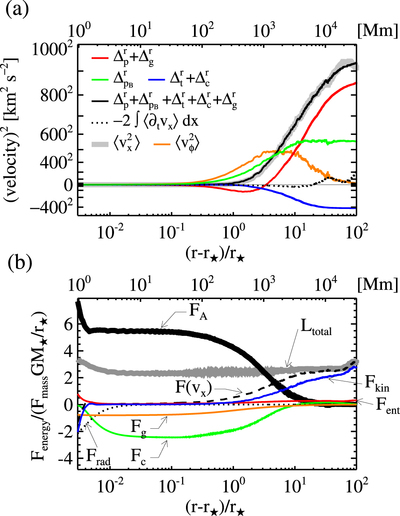Image Details

Choose export citation format:Nonlinear Alfvén Wave Model of Stellar Coronae and Winds from the Sun to M Dwarfs

• Authors: Takahito Sakaue, and Kazunari Shibata

2021 The Astrophysical Journal Letters 906 L13.

• Provider: AAS Journals

Caption: Figure 3.

Momentum and energy conservations in the stellar wind of the M3.5 dwarf. In panel (a), the profile of vx2 (thick gray line) is compared to the contributions by magnetic pressure acceleration ﹩{{\rm{\Delta }}}_{{p}_{B}}^{r}﹩ (green line), sum of plasma pressure ﹩{{\rm{\Delta }}}_{p}^{r}﹩, and gravitational acceleration ﹩{{\rm{\Delta }}}_{g}^{r}﹩ (red line), and sum of centrifugal force ﹩{{\rm{\Delta }}}_{c}^{r}﹩ and magnetic tension force ﹩{{\rm{\Delta }}}_{t}^{r}﹩. The orange line shows the profile of the square amplitude of the Alfvén wave. Panel (b) shows the energy fluxes normalized by FmassGM/r, where Fmass is the mass flux and ﹩{F}_{\mathrm{mass}}{{GM}}_{\star }/{r}_{\star }\approx 5\times {10}^{3}{r}_{\star }^{2}/({{fr}}^{2})﹩ erg cm−2 s−1. FA, Fkin, Fent, Fg, Frad, Fc, and F(vx) are Alfvén wave energy flux, kientic energy flux, enthalpy flux, gravitational energy flux, heat conduction flux, and the sum of enthalpy flux, kinetic energy flux, and Poynting flux advected with the stellar wind, respectively. Ltotal is the integral constant in Equation (2).